Shortcuts

# Hinge Loss¶

## Module Interface¶

class torchmetrics.HingeLoss(task: Literal['binary', 'multiclass'], num_classes: = None, squared: bool = False, multiclass_mode: Optional[Literal['crammer-singer', 'one-vs-all']] = 'crammer-singer', ignore_index: = None, validate_args: bool = True, **kwargs: Any)[source]

Computes the mean Hinge loss typically used for Support Vector Machines (SVMs).

This function is a simple wrapper to get the task specific versions of this metric, which is done by setting the task argument to either 'binary' or 'multiclass'. See the documentation of BinaryHingeLoss and MulticlassHingeLoss for the specific details of each argument influence and examples.

Legacy Example:
>>> import torch
>>> target = torch.tensor([0, 1, 1])
>>> preds = torch.tensor([0.5, 0.7, 0.1])
>>> hinge(preds, target)
tensor(0.9000)

>>> target = torch.tensor([0, 1, 2])
>>> preds = torch.tensor([[-1.0, 0.9, 0.2], [0.5, -1.1, 0.8], [2.2, -0.5, 0.3]])
>>> hinge(preds, target)
tensor(1.5551)

>>> target = torch.tensor([0, 1, 2])
>>> preds = torch.tensor([[-1.0, 0.9, 0.2], [0.5, -1.1, 0.8], [2.2, -0.5, 0.3]])
>>> hinge = HingeLoss(task="multiclass", num_classes=3, multiclass_mode="one-vs-all")
>>> hinge(preds, target)
tensor([1.3743, 1.1945, 1.2359])


### BinaryHingeLoss¶

class torchmetrics.classification.BinaryHingeLoss(squared=False, ignore_index=None, validate_args=True, **kwargs)[source]

Computes the mean Hinge loss typically used for Support Vector Machines (SVMs) for binary tasks. It is defined as: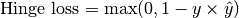Where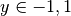is the target, and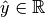is the prediction.

As input to forward and update the metric accepts the following input:

• preds (Tensor): A float tensor of shape (N, ...). Preds should be a tensor containing probabilities or logits for each observation. If preds has values outside [0,1] range we consider the input to be logits and will auto apply sigmoid per element.

• target (Tensor): An int tensor of shape (N, ...). Target should be a tensor containing ground truth labels, and therefore only contain {0,1} values (except if ignore_index is specified). The value 1 always encodes the positive class.

Note

Additional dimension ... will be flattened into the batch dimension.

As output to forward and compute the metric returns the following output:

Parameters

Example

>>> from torchmetrics.classification import BinaryHingeLoss
>>> preds = torch.tensor([0.25, 0.25, 0.55, 0.75, 0.75])
>>> target = torch.tensor([0, 0, 1, 1, 1])
>>> bhl = BinaryHingeLoss()
>>> bhl(preds, target)
tensor(0.6900)
>>> bhl = BinaryHingeLoss(squared=True)
>>> bhl(preds, target)
tensor(0.6905)


Initializes internal Module state, shared by both nn.Module and ScriptModule.

### MulticlassHingeLoss¶

class torchmetrics.classification.MulticlassHingeLoss(num_classes, squared=False, multiclass_mode='crammer-singer', ignore_index=None, validate_args=True, **kwargs)[source]

Computes the mean Hinge loss typically used for Support Vector Machines (SVMs) for multiclass tasks.

The metric can be computed in two ways. Either, the definition by Crammer and Singer is used: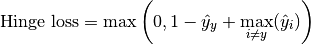Where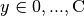is the target class (where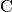is the number of classes), and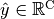is the predicted output per class. Alternatively, the metric can also be computed in one-vs-all approach, where each class is valued against all other classes in a binary fashion.

As input to forward and update the metric accepts the following input:

• preds (Tensor): A float tensor of shape (N, C, ...). Preds should be a tensor containing probabilities or logits for each observation. If preds has values outside [0,1] range we consider the input to be logits and will auto apply softmax per sample.

• target (Tensor): An int tensor of shape (N, ...). Target should be a tensor containing ground truth labels, and therefore only contain values in the [0, n_classes-1] range (except if ignore_index is specified).

Note

Additional dimension ... will be flattened into the batch dimension.

As output to forward and compute the metric returns the following output:

Parameters

Example

>>> from torchmetrics.classification import MulticlassHingeLoss
>>> preds = torch.tensor([[0.25, 0.20, 0.55],
...                       [0.55, 0.05, 0.40],
...                       [0.10, 0.30, 0.60],
...                       [0.90, 0.05, 0.05]])
>>> target = torch.tensor([0, 1, 2, 0])
>>> mchl = MulticlassHingeLoss(num_classes=3)
>>> mchl(preds, target)
tensor(0.9125)
>>> mchl = MulticlassHingeLoss(num_classes=3, squared=True)
>>> mchl(preds, target)
tensor(1.1131)
>>> mchl = MulticlassHingeLoss(num_classes=3, multiclass_mode='one-vs-all')
>>> mchl(preds, target)
tensor([0.8750, 1.1250, 1.1000])


Initializes internal Module state, shared by both nn.Module and ScriptModule.

## Functional Interface¶

torchmetrics.functional.hinge_loss(preds, target, task, num_classes=None, squared=False, multiclass_mode='crammer-singer', ignore_index=None, validate_args=True)[source]

Computes the mean Hinge loss typically used for Support Vector Machines (SVMs).

This function is a simple wrapper to get the task specific versions of this metric, which is done by setting the task argument to either 'binary' or 'multiclass'. See the documentation of binary_hinge_loss() and multiclass_hinge_loss() for the specific details of each argument influence and examples.

Legacy Example:
>>> import torch
>>> target = torch.tensor([0, 1, 1])
>>> preds = torch.tensor([0.5, 0.7, 0.1])
tensor(0.9000)

>>> target = torch.tensor([0, 1, 2])
>>> preds = torch.tensor([[-1.0, 0.9, 0.2], [0.5, -1.1, 0.8], [2.2, -0.5, 0.3]])
tensor(1.5551)

>>> target = torch.tensor([0, 1, 2])
>>> preds = torch.tensor([[-1.0, 0.9, 0.2], [0.5, -1.1, 0.8], [2.2, -0.5, 0.3]])
>>> hinge_loss(preds, target, task="multiclass", num_classes=3, multiclass_mode="one-vs-all")
tensor([1.3743, 1.1945, 1.2359])

Return type

Tensor

### binary_hinge_loss¶

torchmetrics.functional.classification.binary_hinge_loss(preds, target, squared=False, ignore_index=None, validate_args=False)[source]

Computes the mean Hinge loss typically used for Support Vector Machines (SVMs) for binary tasks. It is defined as:Whereis the target, andis the prediction.

Accepts the following input tensors:

• preds (float tensor): (N, ...). Preds should be a tensor containing probabilities or logits for each observation. If preds has values outside [0,1] range we consider the input to be logits and will auto apply sigmoid per element.

• target (int tensor): (N, ...). Target should be a tensor containing ground truth labels, and therefore only contain {0,1} values (except if ignore_index is specified). The value 1 always encodes the positive class.

Additional dimension ... will be flattened into the batch dimension.

Parameters

Example

>>> from torchmetrics.functional.classification import binary_hinge_loss
>>> preds = torch.tensor([0.25, 0.25, 0.55, 0.75, 0.75])
>>> target = torch.tensor([0, 0, 1, 1, 1])
>>> binary_hinge_loss(preds, target)
tensor(0.6900)
>>> binary_hinge_loss(preds, target, squared=True)
tensor(0.6905)

Return type

Tensor

### multiclass_hinge_loss¶

torchmetrics.functional.classification.multiclass_hinge_loss(preds, target, num_classes, squared=False, multiclass_mode='crammer-singer', ignore_index=None, validate_args=False)[source]

Computes the mean Hinge loss typically used for Support Vector Machines (SVMs) for multiclass tasks.

The metric can be computed in two ways. Either, the definition by Crammer and Singer is used:Whereis the target class (whereis the number of classes), andis the predicted output per class. Alternatively, the metric can also be computed in one-vs-all approach, where each class is valued against all other classes in a binary fashion.

Accepts the following input tensors:

• preds (float tensor): (N, C, ...). Preds should be a tensor containing probabilities or logits for each observation. If preds has values outside [0,1] range we consider the input to be logits and will auto apply softmax per sample.

• target (int tensor): (N, ...). Target should be a tensor containing ground truth labels, and therefore only contain values in the [0, n_classes-1] range (except if ignore_index is specified).

Additional dimension ... will be flattened into the batch dimension.

Parameters

Example

>>> from torchmetrics.functional.classification import multiclass_hinge_loss
>>> preds = torch.tensor([[0.25, 0.20, 0.55],
...                       [0.55, 0.05, 0.40],
...                       [0.10, 0.30, 0.60],
...                       [0.90, 0.05, 0.05]])
>>> target = torch.tensor([0, 1, 2, 0])
>>> multiclass_hinge_loss(preds, target, num_classes=3)
tensor(0.9125)
>>> multiclass_hinge_loss(preds, target, num_classes=3, squared=True)
tensor(1.1131)
>>> multiclass_hinge_loss(preds, target, num_classes=3, multiclass_mode='one-vs-all')
tensor([0.8750, 1.1250, 1.1000])

Return type

Tensor

© Copyright Copyright (c) 2020-2023, Lightning-AI et al... Revision 6853c553.

Built with Sphinx using a theme provided by Read the Docs.
Versions
latest
stable
v0.11.2
v0.11.1
v0.11.0
v0.10.3
v0.10.2
v0.10.1
v0.10.0
v0.9.3
v0.9.2
v0.9.1
v0.9.0
v0.8.2
v0.8.1
v0.8.0
v0.7.3
v0.7.2
v0.7.1
v0.7.0
v0.6.2
v0.6.1
v0.6.0
v0.5.1
v0.5.0
v0.4.1
v0.4.0
v0.3.2
v0.3.1
v0.3.0
v0.2.0
v0.1.0